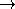## SortedMatrixSearchTraitsadvanced### Definition

The concept SortedMatrixSearchTraits defines types and operations needed to compute the smallest entry in a set of sorted matrices that fulfills a certain feasibility criterion using the function sorted_matrix_search.

### Types

 SortedMatrixSearchTraits::Matrix The class used for representing matrices. It has to be a model for BasicMatrix.

 typedef Matrix::Value Value; The class used for representing the matrix elements.

 SortedMatrixSearchTraits::Compare_strictly An adaptable binary function class: Value  × Valuebool defining a non-reflexive total order on Value. This determines the direction of the search. SortedMatrixSearchTraits::Compare_non_strictly An adaptable binary function class: Value  × Valuebool defining the reflexive total order on Value corresponding to Compare_strictly.

### Operations

 Compare_strictly t.compare_strictly () const returns the Compare_strictly object to be used for the search. Compare_non_strictly t.compare_non_strictly () const returns the Compare_non_strictly object to be used for the search. bool t.is_feasible ( const Value& a) The predicate to determine whether an element a is feasible. It has to be monotone in the sense that compare( a, b) and is_feasible( a) imply is_feasible( b).

### Has Modelsadvanced